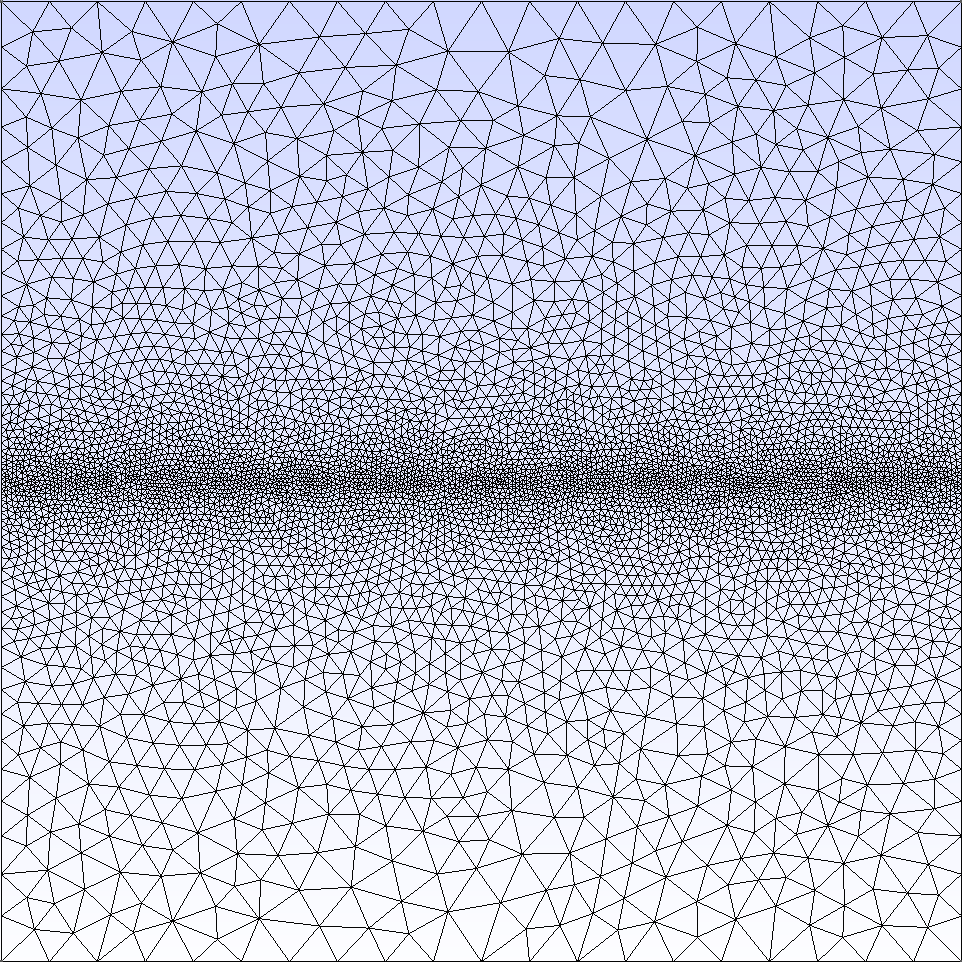Python wrappers to gmsh files with object-oriented syntax

# py2gmsh

Python wrappers to create gmsh files with object-oriented syntax.

The wrappers are made closest to actual gmsh syntax for .geo files, with the addition of convenience tools, object-oriented syntax for easy manipulation and extra functionalities.

## Installation

When pip is present in your python installation, simply:

pip install py2gmsh


## Usage

### Creating a simple geo file

The following example shows how a simple geometry can created using a syntax close to the one used in .geo files

from py2gmsh import (Mesh, Entity, Field)

# create Mesh class instance
my_mesh = Mesh()

# create points
p1 = Entity.Point([0., 0., 0.])
#create more points
p2 = Entity.Point([1., 0., 0.])
p3 = Entity.Point([1., 1., 0.])
# entities can also directly be added to a mesh:
p4 = Entity.Point([0., 1., 0.], mesh=my_mesh)

# create curves
l1 = Entity.Curve([p1, p2])
l2 = Entity.Curve([p2, p3])
l3 = Entity.Curve([p3, p4])
l4 = Entity.Curve([p4, p1])
# entities can also be added in a batch

# create curveloop
ll1 = Entity.CurveLoop([l1, l2, l3, l4], mesh=my_mesh)

# create surface
s1 = Entity.PlaneSurface([ll1], mesh=my_mesh)

# create fields
f1 = Field.MathEval(mesh=my_mesh)
he = 0.005
he=he)
# create minimum field
fmin = Field.Min(mesh=my_mesh)
fmin.FieldsList = [f1]  # could add more fields in the list if necessary

# set the background field as minimum field
my_mesh.setBackgroundField(fmin)

# set max element size
my_mesh.Options.Mesh.CharacteristicLengthMax = 0.1

my_mesh.Coherence = True

# write the geofile
my_mesh.writeGeo('my_mesh.geo')


The .geo file my_mesh.geo can be opened directly in the gmsh GUI for visualisation and meshing. Alternatively, running gmsh to create a .msh file gives the following result for my_mesh.msh:

>> gmsh my_mesh.geo -2 -o my_mesh.msh(!) for Fields using NodesList, VerticesList, EdgesList, FacesList, RegionsList, or FieldsList, the lists must be a list of Entity instances, not of the entity numbers, e.g. f2.NodesList = [p1, p2, p3]. Fields using IField, FieldX, FieldY, FieldZ must also point to a field instance, not its number, e.g. f2.IField = f1.

### Using Physical Groups

Physical groups are used to tag certain entities with a group number and name (optional)

# creating physical groups and associating them with a mesh instance
g1 = Entity.PhysicalGroup(nb=1, name='group1')
g2 = Entity.PhysicalGroup(nb=2, name='group2')

# adding existing entities to different physical groups

# write the geofile after changes
mesh.writeGeo('my_mesh.geo')


### Modifying general mesh options

All gmsh options (General, Geometry, Mesh) can be written with the same syntax as writing directly in a geofile. The full list of options available is in py2gmsh/Options.py

# mesh options
my_mesh.options.Mesh.Algorithm = ...
my_mesh.options.Mesh.Format = ...
# general options
my_mesh.options.General.Color = ...
my_mesh.options.Geometry.OffsetX = ...
# geometry options
my_mesh.options.Geometry.Tolerance = ...

# write the geofile after changes
mesh.writeGeo('my_mesh.geo')


### Accessing entities from mesh instance

my_mesh.points  # <-- returns Point instance number 4
my_mesh.getPointsFromIndex(4)  # <-- same as above
my_mesh.getPointsFromIndex([1, 2, 3, 4])  # <-- returns list of Point instances
# other functions
my_mesh.getCurvesFromIndex(...)
my_mesh.getSurfacesFromIndex(...)
my_mesh.getSurfaceLoopsFromIndex(...)
my_mesh.getVolumesFromIndex(...)
my_mesh.getFieldsFromIndex(...)
my_mesh.getGroupsFromIndex(...)


This can be used to create other entities, such as:

ll1 = Entity.CurveLoop(my_mesh.getCurvesFromIndex([1,2,3,4]))


### Converting a geometry object to a Mesh instance

Certain objects can be directly converted to a py2gmsh.Mesh.Mesh instance. This has been used to convert geometries using the syntax of https://github.com/erdc/proteus domains for example.

from py2gmsh import geometry2gmsh

my_mesh = geometry2mesh(my_geometry)


The geometry variable my_geometry must be an object (e.g. class) containing the following attributes:

entity shape opt type
vertices (np, 3) no array of point coordinates
vertexFlags (np) yes array of point physical group numbers
segments (ns, 2) yes array of curves
segmentFlags (ns) yes array of segment physical group numbers
facets (nf, nsf, npf) yes array of surfaces (loop of point numbers)
facetFlags (nf) yes array of facets physical groups
volumes (nv, nsv, nfv) yes array of volumes (list of facets)
regionFlags (nv) yes array of volume physical group numbers
boundaryTags dict yes dictionary of physical groups {'name': number}
holes_ind (nh) yes list of holes index (facets in 2D / volumes in 3D)

np: number of points; ns: number of segments; nf: number of facets; nsf: number of subfacets; npf: number of points per facet; nv: number of volumes; nsv: number of subvolumes; nfv: number of facets per volume; nh: number of holes

opt: optional. Optional argument can be empty (e.g. empty list) but must be present in the geometry object.

## Project details

This version4.2.3.14.2.3.03.0.6.33.0.6.23.0.6.13.0.6.00.1.20.1.10.1

Uploaded source
Uploaded py3